## Countifs with OR logic for multiple criteria in Excel

Normally, you can use the COUNTIFS function to count cells based on one or more conditions with AND logic in Excel. Have you ever suffered a situation where you need to count more than one value from a single column or a range of cells? This means to count with multiple conditions and OR logic. In this case, you can apply the SUM and COUNTIFS functions together, or use the SUMPRODUCT function.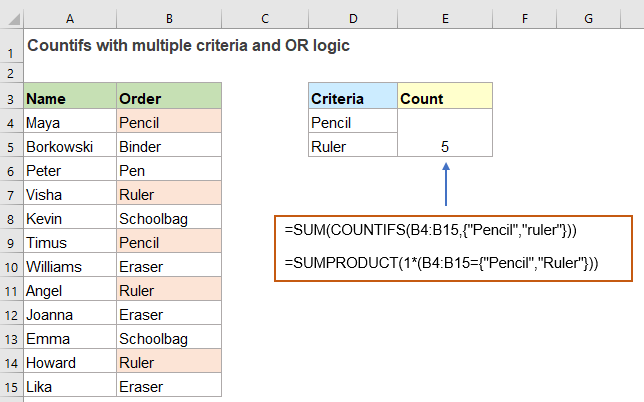#### Count cells with OR conditions in Excel

For example, I have a range of data as below screenshot shown, now, I want to count the number of the product which is “Pencil” or “Ruler”, here, I will discuss two formulas for solving this task in Excel.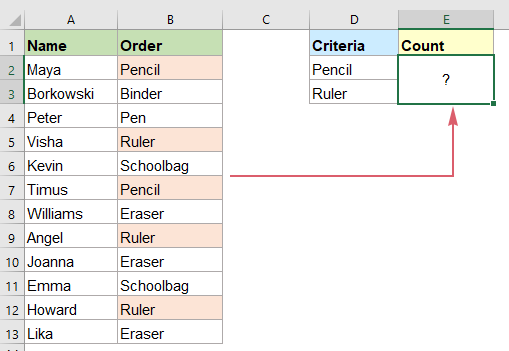##### Count cells with OR conditions by using SUM and COUNTIFS functions

In Excel, to count with multiple OR conditions, you can use the SUM and COUNTIFS functions with an array constant, the generic syntax is:

=SUM(COUNTIF(range, {criterion1, criterion2, criterion3, …}))
• range: The data range contains the criteria where you count cells from;
• criterion1, criterion2, criterion3…: The conditions you want to count cells based on.

To count the number of products which are “Pencil” or “Ruler”, please copy or enter the below formula into a blank cell, and then, press Enter key to get the result:

=SUM(COUNTIFS(B2:B13,{"Pencil","Ruler"}))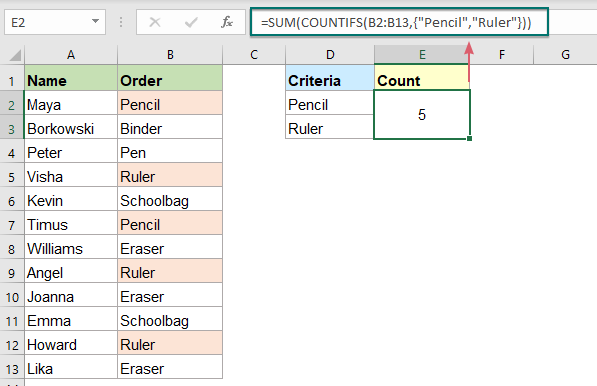##### Explanation of the formula:

=SUM(COUNTIFS(B2:B13,{"Pencil","Ruler"}))

• {"Pencil","Ruler"}: First, you should package all conditions in an array constant like this: {"Pencil","Ruler"}, separate the items by commas.
• COUNTIFS(B2:B13,{"Pencil","Ruler"}): This COUNTIFS function will get individual counts for “Pencil” and “Ruler”, and you will get the result as this: {2,3}.
• SUM(COUNTIFS(B2:B13,{"Pencil","Ruler"}))=SUM({2,3}): Finally, this SUM function sums all items in the array and returns the result: 5.

Tips: You can also use cell references for criteria, please apply the below array formula, and then press Ctrl + Shift + Enter keys together to get the correct result:

=SUM(COUNTIF(B2:B13,D2:D3))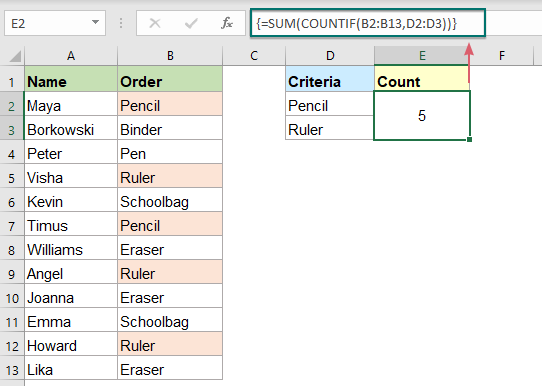##### Count cells with OR conditions by using SUMPRODUCT function

Here is another formula which is created by the SUMPRODUCT function that can help to count cells with OR logic as well. The generic syntax is:

=SUMPRODUCT(1*(range ={criterion1, criterion2, criterion3, …}))
• range: The data range contains the criteria where you count cells from;
• criterion1, criterion2, criterion3…: The conditions you want to count cells based on.

Please copy or enter the following formula into a blank cell, and press Enter key to return the result:

=SUMPRODUCT(1*(B2:B13={"Pencil","Ruler"}))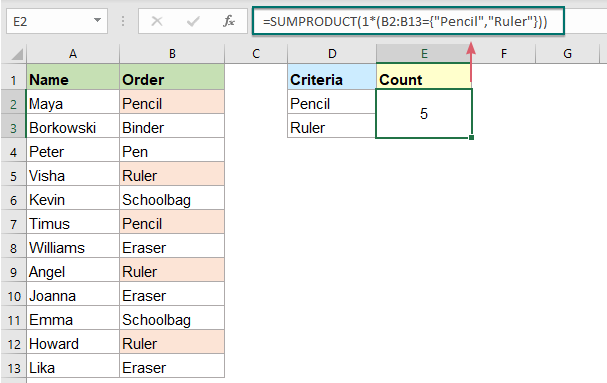##### Explanation of the formula:

=SUMPRODUCT(1*(B2:B13={"Pencil","Ruler"}))

• B2:B13={"Pencil","Ruler"}: This expression compares each criterion “Pencil”, “Ruler” with range cell B2:B13. If the criterion is met, it returns a TRUE, otherwise, a FALSE will display, you will get the result as this: {TRUE,FALSE;FALSE,FALSE;FALSE,FALSE;FALSE,TRUE;FALSE,FALSE;TRUE,FALSE;FALSE,FALSE;FALSE,TRUE;FALSE,FALSE;FALSE,FALSE;FALSE,TRUE;FALSE,FALSE}.
• 1*(B2:B13={"Pencil","Ruler"}): Multiplication converts the logical values – TRUE and FALSE to 1 and 0, so it will get the result like this: {1,0;0,0;0,0;0,1;0,0;1,0;0,0;0,1;0,0;0,0;0,1;0,0}.
• SUMPRODUCT(1*(B2:B13={"Pencil","Ruler"}))= SUMPRODUCT({1,0;0,0;0,0;0,1;0,0;1,0;0,0;0,1;0,0;0,0;0,1;0,0}): At last, the SUMPRODUCT function adds up all numbers in the array to get the result: 5.

#### Count cells with multiple sets of OR conditions in Excel

Sometimes, you may need to count the cells with two or more sets of OR conditions, in this case, you can use either SUM and COUNTIFS with an array constant or SUMPRODUCT with ISNUMBER MATCH functions.

##### Count cells with two sets of OR conditions by using SUM and COUNTIFS functions

To deal with only two sets of OR criteria, you just need to add another array constant to the COUNTIFS formula.

For example, I have the data range as below screenshot shown, now, I want to count the people who ordered “Pencil” or “Ruler” and the Amount is either <100 or >200.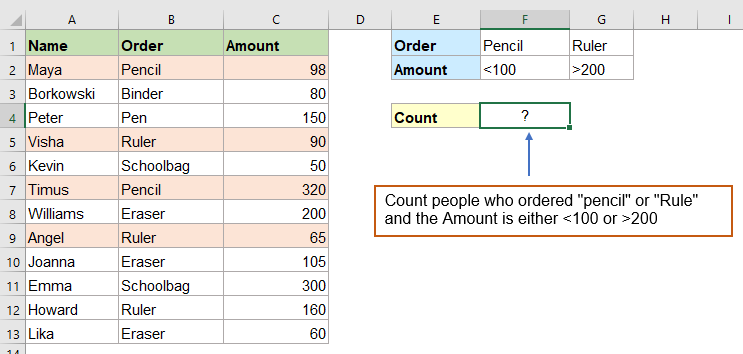Please enter or copy the following formula into a cell, and then press Enter key to get the result, see screenshot:

=SUM(COUNTIFS(B2:B13,{"Pencil","Ruler"},C2:C13,{"<100";">200"}))

Note: In the formula, you should use a semicolon for the second array constant, which creates a vertical array.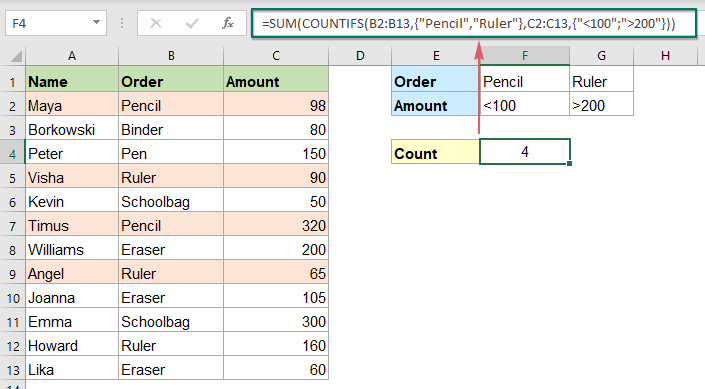##### Count cells with multiple sets of OR conditions by using SUMPRODUCT function

The above formula only works for two sets of OR criteria, if you need to count with more criteria, a complex SUMPRODUCT formula together with ISNUMBER MATCH functions can help you.

Take the below data for example, to count the people who ordered "Pencil" or "Ruler" and the Status is either "Delivered" or "In transit" and signed by "Bob" or "Eko", you should apply a complex formula.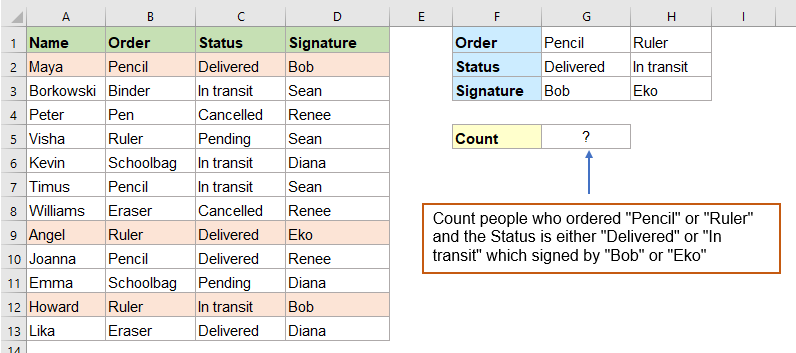Please copy or enter the below formula into a blank cell, and then press Enter key to get the calculation, see screenshot:

=SUMPRODUCT(ISNUMBER(MATCH(B2:B13,{"Pencil","Ruler"},0))*ISNUMBER(MATCH(C2:C13,{"Delivered","In transit"},0))*ISNUMBER(MATCH(D2:D13,{"Bob","Eko"},0)))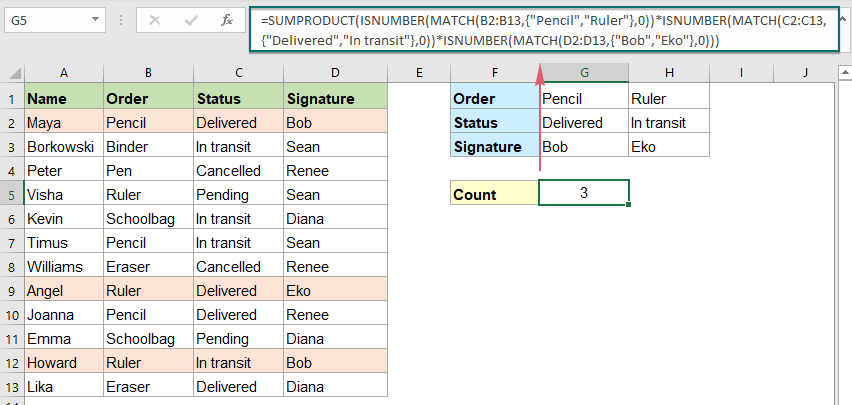##### Explanation of the formula:

=SUMPRODUCT(ISNUMBER(MATCH(B2:B13,{"Pencil","Ruler"},0))*ISNUMBER(MATCH(C2:C13,{"Delivered","In transit"},0))*ISNUMBER(MATCH(D2:D13,{"Bob","Eko"},0)))

ISNUMBER(MATCH(B2:B13,{"Pencil","Ruler"},0)):

• MATCH(B2:B13,{"Pencil","Ruler"},0): This MATCH function is used to compare each cell in range B2:B13 with the corresponding array constant. If the match is found, it returns a relative position of the value in the array, otherwise an error value displayed. So, you will get the array list as this: {1;#N/A;#N/A;2;#N/A;1;#N/A;2;1;#N/A;2;#N/A}.
• ISNUMBER(MATCH(B2:B13,{"Pencil","Ruler"},0))= ISNUMBER({1;#N/A;#N/A;2;#N/A;1;#N/A;2;1;#N/A;2;#N/A}):The ISNUMBER function converts the numbers to TRUEs and error values to FALSEs as this: {TRUE;FALSE;FALSE;TRUE;FALSE;TRUE;FALSE;TRUE;TRUE;FALSE;TRUE;FALSE}.

The above logic can also be applied to the second and third ISNUMBER expressions.

SUMPRODUCT(ISNUMBER(MATCH(B2:B13,{"Pencil","Ruler"},0))*ISNUMBER(MATCH(C2:C13,{"Delivered","In transit"},0))*ISNUMBER(MATCH(D2:D13,{"Bob","Eko"},0))):

• Then, these three arrays are multiplied together inside SUMPRODUCT, which automatically converts TRUE and FALSE values to 1s and 0s as part of the math operation like this: SUMPRODUCT({1;0;0;1;0;1;0;1;1;0;1;0}*{1;1;0;0;1;1;0;1;1;0;1;1} *{1;0;0;0;0;0;0;1;0;0;1;0})=SUMPRODUCT({1;0;0;0;0;0;0;1;0;0;1;0}).
• At last, the SUMPRODUCT function sums all numbers in the array to get the result: 3.

#### Relative function used:

• SUM:
• The Excel SUM function returns the sum of values supplied.
• COUNTIF:
• The COUNTIF function is a statistical function in Excel which is used to count the number of cells that meet a criterion.
• SUMPRODUCT:
• The SUMPRODUCT function can be used to multiply two or more columns or arrays together, and then get the sum of products.
• ISNUMBER:
• The Excel ISNUMBER function returns TRUE when a cell contains a number, and FALSE if not.
• MATCH:
• The Microsoft Excel MATCH function searches for a specific value in a range of cells, and returns the relative position of this value.

#### More articles:

• Count Unique Numeric Values Based On Criteria
• In Excel worksheet, you may suffer from a problem that count the number of unique numeric values based on a specific condition. For example, how can I count the unique Qty values of the product “T-shirt” from the report as below screenshot shown? In this article, I will show some formulas to achieve this task in Excel.
• Count Number Of Rows With Multiple OR Criteria
• To count the number of rows with multiple criteria in different columns, with OR logic, the SUMPRODUCT function can help you. For example, I have a product report as below screenshot shown, now, I want to count the rows where the product is “T-shirt” or the color is “Black”. How to deal with this task in Excel?

### The Best Office Productivity Tools

#### Kutools for Excel - Helps You To Stand Out From Crowd

 Popular Features: Find/Highlight/Identify Duplicates  |  Delete Blank Rows  |  Combine Columns or Cells without Losing Data  |  Round without Formula ... Super VLookup: Multiple Criteria  |  Multiple Value  |  Across Multi-Sheets  |  Fuzzy Lookup... Adv. Drop-down List: Easy Drop Down List  |  Dependent Drop Down List  |  Multi-select Drop Down List... Column Manager: Add a Specific Number of Columns  |  Move Columns  |  Toggle Visibility Status of Hidden Columns  |  Compare Columns to Select Same & Different Cells ... Featured Features: Grid Focus  |  Design View  |  Big Formula Bar  |  Workbook & Sheet Manager | Resource Library (Auto Text)  |  Date Picker  |  Combine Worksheets  |  Encrypt/Decrypt Cells  |  Send Emails by List  |  Super Filter  |  Special Filter (filter bold/italic/strikethrough...) ... Top 15 Toolsets:  12 Text Tools (Add Text, Remove Characters ...)  |  50+ Chart Types (Gantt Chart ...)  |  40+ Practical Formulas (Calculate age based on birthday ...)  |  19 Insertion Tools (Insert QR Code, Insert Picture from Path ...)  |  12 Conversion Tools (Numbers to Words, Currency Conversion ...)  |  7 Merge & Split Tools (Advanced Combine Rows, Split Cells ...)  |  ... and more

Kutools for Excel Boasts Over 300 Features, Ensuring That What You Need is Just A Click Away...

Supports Office/Excel 2007-2021 and 365   |   Available in 44 Languages   |   Easy to Uninstall Completely#### Office Tab - Enable Tabbed Reading and Editing in Microsoft Office (include Excel)

• One second to switch between dozens of open documents!
• Reduce hundreds of mouse clicks for you every day, say goodbye to mouse hand.
• Increases your productivity by 50% when viewing and editing multiple documents.
• Brings Efficient Tabs to Office (include Excel), Just Like Chrome, Edge and Firefox.